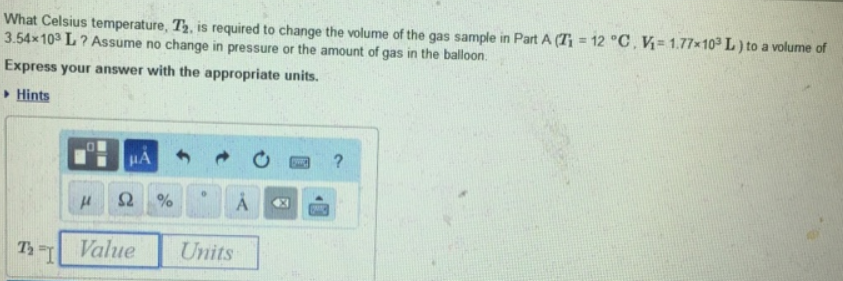Chemistry Practice Problems Chemistry Gas Laws Practice Problems Solution: What Celsius temperature, T2, is required to chang...

# Solution: What Celsius temperature, T2, is required to change the volume of the gas sample in Part A (T1 = 12 °C, V1 = 1 77 x 103 L) to a volume of 3.54 x 103 L? Assume no change in pressure or the amount of gas in the balloon. Express your answer with the appropriate units.

###### Problem

What Celsius temperature, T2, is required to change the volume of the gas sample in Part A (T1 = 12 °C, V1 = 1 77 x 103 L) to a volume of 3.54 x 103 L? Assume no change in pressure or the amount of gas in the balloon.View Complete Written Solution

Chemistry Gas Laws

Chemistry Gas Laws

#### Q. A cylinder with a moveable piston contains 0.95 mol of gas and has a volume of 343 mL. Part AWhat will its volume be if an additional 0.17 mol of gas ...

Solved • Wed Aug 08 2018 14:16:04 GMT-0400 (EDT)

Chemistry Gas Laws

#### Q. Enter your answer in the provided box.Under constant-pressure conditions a sample of hydrogen gas initially at 73.00 °C and 9.40 L is cooled until its...

Solved • Wed Aug 01 2018 14:19:25 GMT-0400 (EDT)

Chemistry Gas Laws

#### Q. Enter your answer in the provided box.A gas at 693 mmHg and 31 °C occupies a volume of 7.04 L. Calculate its volume at STP.

Solved • Wed Aug 01 2018 12:40:37 GMT-0400 (EDT)

Chemistry Gas Laws

#### Q. Part AA sample of a gas is in a sealed container. The pressure of the gas is 625 torr, and the temperature is 21 °C. If the temperature changes to 75 ...

Solved • Tue Jul 31 2018 15:02:41 GMT-0400 (EDT)